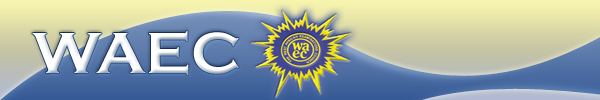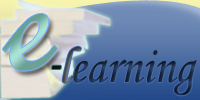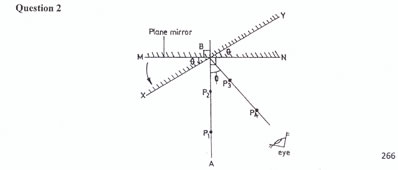Physics Paper 1, May/June. 2010
 Questions: 1 2 3 4 5 6 Main
General Comments
Weakness/Remedies
Strength

Alternative b
Question 5

(a)  Diagram1.  Fix the drawing paper provided on the cellotex board.
2.  Place the plane mirror vertically, along its breadth, on the drawing paper.  Trace its outline MN, Remove the mirror
3.  Mark the middle, B of the outline. Draw a normal AB to meet the outline at B.
4.  Draw another line XY through B such that it makes an angle i = 10o at B, with the outline of the mirror.
5.  Fix two pins, P1 and P2, on AB. Replace the mirror on its outline. Rotate the mirror gently until it tests on the new line XY.  Ensure that the position of B on the  outline  is maintained
6.  Locate the images of P1 and P2 in the mirror. Fix two other pins P3 and P4 such that they appear to be a straight line with the images of P1 and P2.
7.  Remove the mirror and the pins P3 and P4
8.  Draw a line to join the pin holes of P3 and P4. Produce the line to meet MN at B as shown in the diagram above.
9. Measure and record ф.
10. Repeat the procedure for four other values of θ = 15o, 20o, 25o and 30o.  In each case, measure and record the corresponding value of ф.
11. Tabulate your readings.
12. Plot a graph with ф on the vertical axis and θ on the horizontal axis.
13. Determine the slope, s, of the graph.
14. State two precautions taken to obtain accurate results.

(b)   (i)   State the laws of reflection of light.

(ii)   List four application of plane mirrors

-

_____________________________________________________________________________________________________
Observation

This question on rotation of mirror was the least popular among the candidates.  It was however satisfactorily answered by the few candidates that attempted it.  The observation was well handled.  The candidates were able to plot the graph of ф against θ, determine slope of the graph and state precautions correctly in acceptable language.  Some candidates however, failed to attach their traces to their answer booklet as demanded in the question.  In part b, few candidates could not state the laws of reflection but were able to list applications of plane mirror.

The expected responses were as follows:

In part (a) the candidates were required to perform the following tasks to score good points

-     Draw five complete traces showing incident ray reflected ray angles ф and θ.
-     Measure and record in degrees five values each of θ and ф in trend.
Trend:  As θ increases, ф increases ф =   2o.
-     Record data in composite table showing θ and ф.
-     Indicate the graph axes, select reasonable scales, plot the five points and line of best fit
through the points.
-     Determine the slope of the graph using large right-angled triangle
-     State any two of the following precautions in acceptable form of speech.
-     Ensured pins were vertical

-     Avoided parallax error when reading protractor.
-     Evidence of pin spacing
-     Evidence of sharp pencil/neat traces.

The expected responses in part (b) were as follow:

(i) Laws of reflection

-    angle of incidence (i) = angle of reflection (i)
-    incident ray, reflected  ray and normal (at a point of incidence) all lie in the same
plane.

(ii) Applications of plane mirrors

-    Periscope
-    Looking/dressing mirror/glass
-    Kaleidoscope
-    Sextant.

 Powered by Sidmach Technologies(Nigeria) Limited . Copyright © 2015 The West African Examinations Council. All rights reserved.